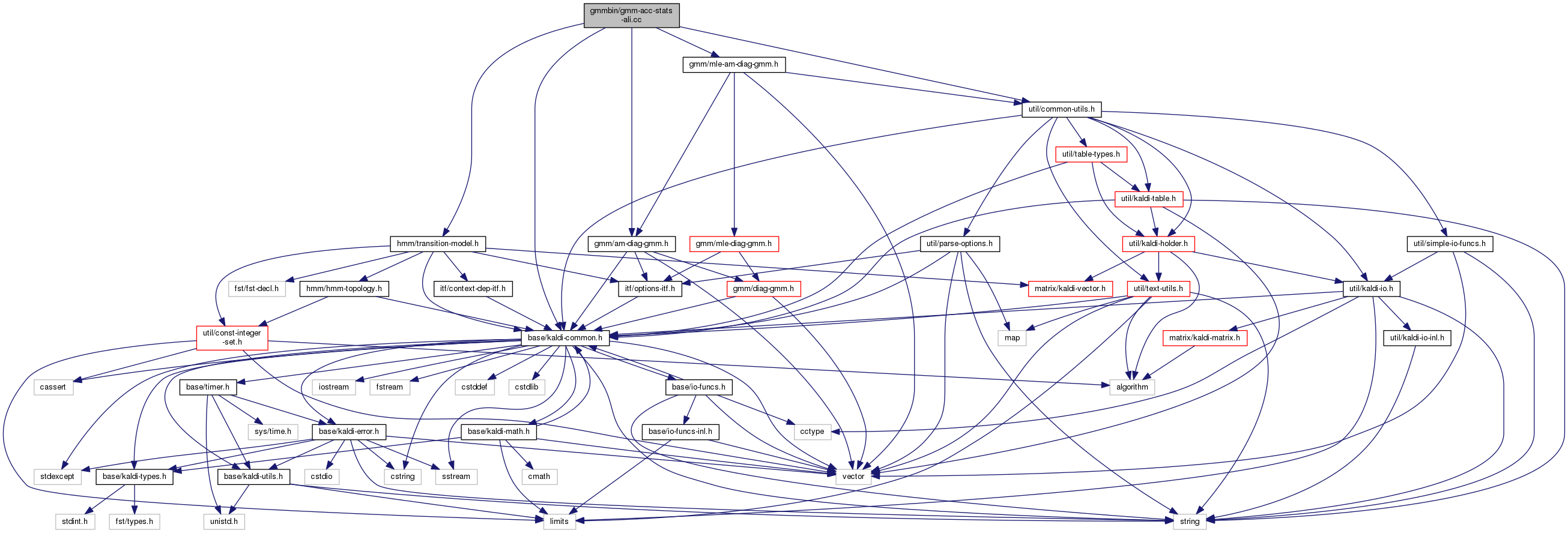gmm-acc-stats-ali.cc File Reference
`#include "base/kaldi-common.h"`
`#include "util/common-utils.h"`
`#include "gmm/am-diag-gmm.h"`
`#include "hmm/transition-model.h"`
`#include "gmm/mle-am-diag-gmm.h"`
Include dependency graph for gmm-acc-stats-ali.cc:Go to the source code of this file.

## Functions

int main (int argc, char *argv[])

## ◆ main()

 int main ( int argc, char * argv[] )

Definition at line 30 of file gmm-acc-stats-ali.cc.

30  {
31  using namespace kaldi;
32  typedef kaldi::int32 int32;
33  try {
34  const char *usage =
35  "Accumulate stats for GMM training.\n"
36  "Usage: gmm-acc-stats-ali [options] <model-in> <feature-rspecifier> "
37  "<alignments-rspecifier> <stats-out>\n"
38  "e.g.:\n gmm-acc-stats-ali 1.mdl scp:train.scp ark:1.ali 1.acc\n";
39
40  ParseOptions po(usage);
41  bool binary = true;
42  po.Register("binary", &binary, "Write output in binary mode");
44
45  if (po.NumArgs() != 4) {
46  po.PrintUsage();
47  exit(1);
48  }
49
50  std::string model_filename = po.GetArg(1),
51  feature_rspecifier = po.GetArg(2),
52  alignments_rspecifier = po.GetArg(3),
53  accs_wxfilename = po.GetArg(4);
54
55  AmDiagGmm am_gmm;
56  TransitionModel trans_model;
57  {
58  bool binary;
59  Input ki(model_filename, &binary);
62  }
63
64  Vector<double> transition_accs;
65  trans_model.InitStats(&transition_accs);
66  AccumAmDiagGmm gmm_accs;
67  gmm_accs.Init(am_gmm, kGmmAll);
68
69  double tot_like = 0.0;
70  kaldi::int64 tot_t = 0;
71
74
75  int32 num_done = 0, num_err = 0;
79  KALDI_WARN << "No alignment for utterance " << key;
80  num_err++;
81  } else {
82  const Matrix<BaseFloat> &mat = feature_reader.Value();
83  const std::vector<int32> &alignment = alignments_reader.Value(key);
84
85  if (alignment.size() != mat.NumRows()) {
86  KALDI_WARN << "Alignments has wrong size " << (alignment.size())
87  << " vs. " << (mat.NumRows());
88  num_err++;
89  continue;
90  }
91
92  num_done++;
93  BaseFloat tot_like_this_file = 0.0;
94
95  for (size_t i = 0; i < alignment.size(); i++) {
96  int32 tid = alignment[i], // transition identifier.
97  pdf_id = trans_model.TransitionIdToPdf(tid);
98  trans_model.Accumulate(1.0, tid, &transition_accs);
99  tot_like_this_file += gmm_accs.AccumulateForGmm(am_gmm, mat.Row(i),
100  pdf_id, 1.0);
101  }
102  tot_like += tot_like_this_file;
103  tot_t += alignment.size();
104  if (num_done % 50 == 0) {
105  KALDI_LOG << "Processed " << num_done << " utterances; for utterance "
106  << key << " avg. like is "
107  << (tot_like_this_file/alignment.size())
108  << " over " << alignment.size() <<" frames.";
109  }
110  }
111  }
112  KALDI_LOG << "Done " << num_done << " files, " << num_err
113  << " with errors.";
114
115  KALDI_LOG << "Overall avg like per frame (Gaussian only) = "
116  << (tot_like/tot_t) << " over " << tot_t << " frames.";
117
118  {
119  Output ko(accs_wxfilename, binary);
120  transition_accs.Write(ko.Stream(), binary);
121  gmm_accs.Write(ko.Stream(), binary);
122  }
123  KALDI_LOG << "Written accs.";
124  if (num_done != 0)
125  return 0;
126  else
127  return 1;
128  } catch(const std::exception &e) {
129  std::cerr << e.what();
130  return -1;
131  }
132 }
This code computes Goodness of Pronunciation (GOP) and extracts phone-level pronunciation feature for...
Definition: chain.dox:20
void Write(std::ostream &Out, bool binary) const
Writes to C++ stream (option to write in binary).
BaseFloat AccumulateForGmm(const AmDiagGmm &model, const VectorBase< BaseFloat > &data, int32 gmm_index, BaseFloat weight)
Accumulate stats for a single GMM in the model; returns log likelihood.
kaldi::int32 int32
int32 TransitionIdToPdf(int32 trans_id) const
Allows random access to a collection of objects in an archive or script file; see The Table concept...
Definition: kaldi-table.h:233
float BaseFloat
Definition: kaldi-types.h:29
void InitStats(Vector< double > *stats) const
The class ParseOptions is for parsing command-line options; see Parsing command-line options for more...
Definition: parse-options.h:36
const SubVector< Real > Row(MatrixIndexT i) const
Return specific row of matrix [const].
Definition: kaldi-matrix.h:188
void Accumulate(BaseFloat prob, int32 trans_id, Vector< double > *stats) const
A templated class for reading objects sequentially from an archive or script file; see The Table conc...
Definition: kaldi-table.h:287
#define KALDI_WARN
Definition: kaldi-error.h:150
MatrixIndexT NumRows() const
Returns number of rows (or zero for empty matrix).
Definition: kaldi-matrix.h:64
void Write(std::ostream &out_stream, bool binary) const
#define KALDI_LOG
Definition: kaldi-error.h:153